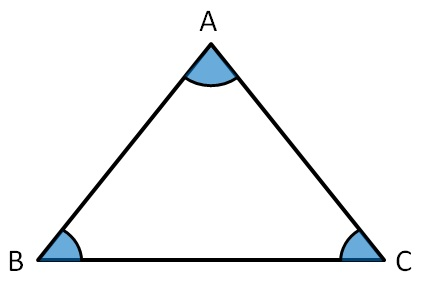Classifying triangles

Chapter 5 Class 6 Understanding Elementary Shapes
Concept wise

A Triangle has 3 sides and 3 angles.

Thus, triangle can be classified on the basis of

• Sides
• AnglesSo, all sides can be equal, or 2 sides can be equal, or all sides might be different.

Similarly, for angles

• All angles can be equal
• Angles can be greater than 90, or less than 90
• Angle can be equal to right angle as well.

Let's learn how we classify them. Click on the next button below.

Learn in your speed, with individual attention - Teachoo Maths 1-on-1 Class